# Algebra II : Multiplying and Dividing Fractions

## Example Questions

← Previous 1 3 4 5 6 7 8 9 10 11

### Example Question #1 : Multiplying And Dividing Fractions

Solve the following equation to find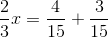Possible Answers: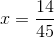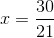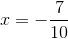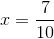Correct answer:Explanation:

The first step in solving this equation is to add the fractions, giving us: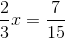To solve for, we need to divide both sides by.

Remember: When we divide a number by a fraction, we "switch" (find the reciprocal) of the fraction and mulitply it to the number.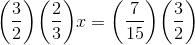The right side of the equation cancels out leavingalone: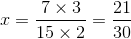Notice: Both the numerator and denominator are divisble byso we can simplify this further.### Example Question #2 : Multiplying And Dividing Fractions

Simplify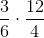.

Possible Answers: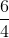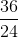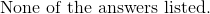Correct answer:Explanation:

The problem can be made easier by first simplifying each fraction: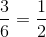and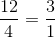This brings our new problem to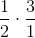.

Now, the numerators are multiplied by each other then the denomenators are multiplied by each other: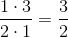.

### Example Question #3 : Multiplying And Dividing Fractions

Simplify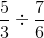.

Possible Answers: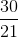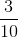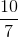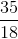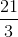Correct answer:Explanation:

To solve, we must turn the division problem into a multiplication problem by "flipping" the second fraction (dividing by a fraction is the same as multiplying by its reciprocal):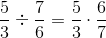.

Then, we multiply the numerators followed by the denomenators: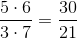.

Lastly, the fraction must be simplified by a factor of 3: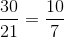, which gives us our final answer.

### Example Question #4 : Multiplying And Dividing Fractions

Multiply: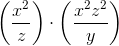Possible Answers: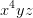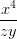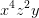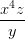Correct answer:Explanation:

To multiply fractions, just multiply the numerators, then the denominators, and then simplify.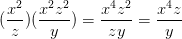### Example Question #5 : Multiplying And Dividing Fractions

Multiply: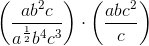Possible Answers: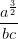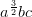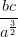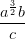Correct answer:Explanation:

To multiply fractions, multiply the numerators and denominators together, then simplify.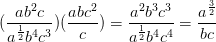### Example Question #6 : Multiplying And Dividing Fractions

Multiply: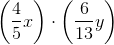Possible Answers: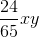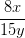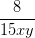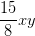Correct answer:Explanation:

Multiply the numerators and denominators. Then, simplify.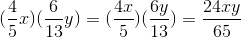### Example Question #7 : Multiplying And Dividing Fractions

Multiply: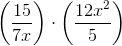Possible Answers: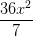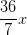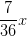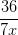Correct answer:Explanation:

Multiply the numerators and denominators, then simplify.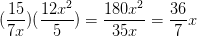### Example Question #8 : Multiplying And Dividing Fractions

Simplify: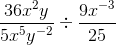Possible Answers: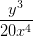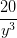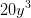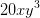Correct answer:Explanation:

In order to divide fractions, you need to multiply the first fraction by the reciprocal of the second one.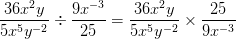Now, multiply the numerators and denominators together, then simplify.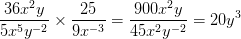### Example Question #9 : Multiplying And Dividing Fractions

Simplify: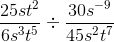Possible Answers: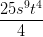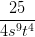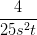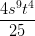Correct answer:Explanation:

To divid fractions, you need to multiply the first fraction by the reciprocal of the second.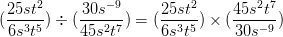Now, multiply the numerators and denominators together, then simplify.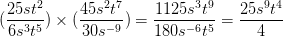### Example Question #181 : Fractions

Simplify: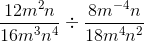Possible Answers: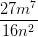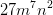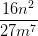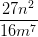Correct answer:Explanation:

To divide fractions, you need to multiply the first fraction by the reciprocal of the second.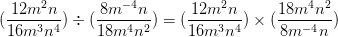Now, multiply the numerators and denominators, then simplify.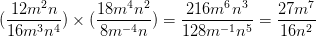← Previous 1 3 4 5 6 7 8 9 10 11

### All Algebra II Resources